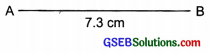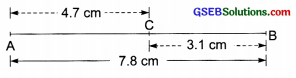# GSEB Solutions Class 6 Maths Chapter 14 Practical Geometry Ex 14.2

Gujarat Board GSEB Textbook Solutions Class 6 Maths Chapter 14 Practical Geometry Ex 14.2 Textbook Questions and Answers.

## Gujarat Board Textbook Solutions Class 6 Maths Chapter 14 Practical Geometry Ex 14.2

Question 1.
Draw a line segment of length 7.3 cm using a ruler.Solution:
Steps of construction:
Step I: Mark a point A.
Step II: Place the zero mark of the ruler against point A.
Step III: Mark a point B at a distance of 7.3 cm from A.
Step IV: Join A and B.
Thus, $$\overline{A B}$$ is the required line segment of length 7.3 cm.
Note: While marking points A and B, we should look straight down at the measuring device. Otherwise, we will get the incorrect length.

Question 2.
Construct a line segment of length 5.6 cm using a ruler and compasses.
Solution:
Steps of construction:
Step I: Draw a line l and mark a point ‘A’ on it.Step II: Place the steel-end of the compasses on the zero mark of the ruler. Open it such that the pencil tip falls on the 5.6 cm mark.
Step III: Without changing the opening of the compasses, place the steel end on ‘A’ and mark an arc to cut l at ‘B’.
Thus, $$\overline{A B}$$ = 5.6 cm is the line segment of the required length.Question 3.
Construct $$\overline{A B}$$ of length 7.8 cm. From this, cut-off $$\overline{A C}$$ of length 4.7 cm. Measure $$\overline{B C}$$
Solution:
Steps of construction:
Step I: Place the zero mark of the ruler at A.
Step II: Mark a point B at a distance of 7.8 cm from A.Step III: Mark another point C between A and B at a distance of 4.7 cm from A such that $$\overline{A C}$$ = 4.7 cm.
Step IV: Measure the line segment $$\overline{B C}$$. We find that $$\overline{B C}$$ =3.1 cm.

Question 4.
Given $$\overline{A B}$$ of length 3.9 cm, construct $$\overline{P Q}$$ such that the length of $$\overline{P Q}$$ is twice that of $$\overline{A B}$$. Verify by measurement.Hint: Construct $$\overline{P X}$$ such that length of $$\overline{P X}$$ = length of $$\overline{A B}$$; then cut-off $$\overline{X Q}$$ such that $$\overline{X Q}$$ also has the length of $$\overline{A B}$$.
Solution:
Steps of construction:
Step I: Draw a line l.
Step II: Draw AB = 3.9 cm.Step III: On line l construct $$\overline{P X}$$ = $$\overline{A B}$$ (= 3.9 cm).
Step IV: Next construct $$\overline{X Q}$$ = $$\overline{A B}$$ (=3.9 cm)
Thus, the lengths of $$\overline{P X}$$ and $$\overline{X Q}$$ are added together to make twice the length of $$\overline{A B}$$
Verification: By measurement, we have:
$$\overline{A B}$$ + $$\overline{A B}$$ = 3.9 cm + 3.9 cm
2 ( $$\overline{A B}$$ ) = 7.8 cm = $$\overline{X Y}$$
Thus, twice of $$\overline{A B}$$ is equal to $$\overline{X Y}$$Question 5.
Given length 7.3 cm and $$\overline{C D}$$ of length 3.4 cm, construct a line segment $$\overline{X Y}$$ such that the length of $$\overline{X Y}$$ is equal to the difference between the lengths of $$\overline{A B}$$ and $$\overline{C D}$$ Verify by measurement.
Solution:
Step I: Draw $$\overline{A B}$$ = 7.3 cm and $$\overline{C D}$$ =3.4 cm.
Step II: Draw a line l and take a point X on it.
Step III: Construct $$\overline{X R}$$ such that length of $$\overline{X R}$$ = length $$\overline{A B}$$ (= 7.3 cm).Step IV: Cut-off $$\overline{RY}$$ = length of $$\overline{C D}$$ (= 3.4 cm) such that the length $$\overline{X Y}$$ = length
of $$\overline{A B}$$ – length of $$\overline{C D}$$.
Verification: By measurement, we have
$$\overline{X Y}$$ = 3.9 cm = 7.3 cm – 3.4 cm
= $$\overline{A B}$$ – $$\overline{C D}$$
Thus, we get $$\overline{X Y}$$ = $$\overline{A B}$$ – $$\overline{C D}$$
Now, the opening of compasses is equal to $$\overline{A B}$$.
Step IV: Draw a line l and mark a point C on it.
Step V: Without changing the openings of the compasses, place the steel end at C and mark a point D on l.
Now, $$\overline{C D}$$ is a copy of $$\overline{A B}$$.